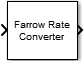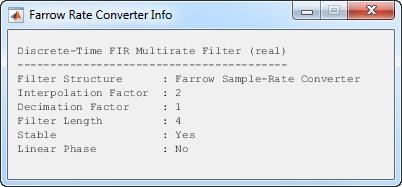# Farrow Rate Converter

Polynomial sample-rate converter with arbitrary conversion factor

## Library

Signal Operations

`dspsigops`

•## Description

The Farrow Rate Converter block converts the sample rate of an input signal using polynomial fit sample-rate conversion. Polynomial-based filters are efficient at implementing fractional sample rate conversion. Farrow structures are implementations of polynomial-based filters. This block uses a Farrow structure to implement arbitrary rate-change factors efficiently. The rate-change factors can be irrational.

The input frame size must be a multiple of the decimation factor of the rate converter. The decimation factor depends on the parameter settings of the block. To determine the decimation factor, in the block dialog box click .

Each column of the input signal is treated as a separate channel. If the input is a two-dimensional signal, the first dimension represents the channel length (or frame size) and the second dimension represents the number of channels. If the input is a one-dimensional signal, then it is interpreted as a single channel. The inputs to the block can be single, double, or fixed-point data type.

## Parameters

### Main

Sample rate of input signal (Hz)

Sample rate of the input signal, specified as a positive scalar in Hz. The input sample rate must be greater than the bandwidth of interest. The default is `48e3`.

Sample rate of output signal (Hz)

Sample rate of the output signal, specified as a positive scalar in Hz. The output sample rate must be higher or lower than the input sample rate. The default is `98e3`.

Tolerance for output sample rate

Maximum allowed tolerance for the output sample rate, specified as a positive scalar in the range [`0` to`0.5`]. The default is `0`.

The actual output sample rate varies but is within the specified range. For example, suppose that you set the Tolerance for output sample rate to 0.01. Then the actual output sample rate is in the range given by sample rate of output signal ± 1%. This flexibility allows for a simpler filter design.

Specification method

Method used to specify the polynomial interpolator coefficients, specified as one of the following:

• `Polynomial order` — Specify the order of the Lagrange interpolation filter polynomial through the Polynomial order parameter.

• `Coefficients` — Specify the polynomial coefficients directly through the Coefficients parameter.

Polynomial order

Order of filter polynomial, specified as a `1`, `2`, `3`, or `4`. The default is `3`. This parameter applies only when you set Specification method to `Polynomial order`.

Coefficients

Filter polynomial coefficients, specified as a real-valued square matrix. The default is `[-1 1; 1 0]`. This property applies only when you set Specification method to `Coefficients`.

View Filter Response

Opens the fvtool and displays the magnitude/phase response of the Farrow Rate Converter. The response is based on the block dialog box parameters. Changes made to these parameters update FVTool.To update the magnitude response while fvtool is running, modify the dialog box parameters and click .

View Info

Display the following information about the Farrow filter system:

• `Filter Structure`

• `Interpolation Factor`

• `Decimation Factor`

• `Filter Length`

• `Stable`

• `Linear Phase`This button brings the functionality of the `info` method into the Simulink® environment.

Simulate using

Type of simulation to run. You can set this parameter to:

• `Code generation` (default)

Simulate model using generated C code. The first time you run a simulation, Simulink generates C code for the block. The C code is reused for subsequent simulations, as long as the model does not change. This option requires additional startup time but provides faster simulation speed than ```Interpreted execution```.

• `Interpreted execution`

Simulate model using the MATLAB®  interpreter. This option shortens startup time but has slower simulation speed than ```Code generation```.

### Data Types

Rounding mode

Rounding mode for fixed-point operations, specified as one of `Ceiling` | `Convergent` | `Floor` | `Nearest` | `Round` | `Zero`. The default is `Floor`. For more information on the rounding modes, see Precision and Range.

This property is not tunable.

Saturate on integer overflow

Overflow action for fixed-point operations, specified as `Wrap` | `Saturate` . The default is `Wrap`. For more details on the overflow action to select, see the 'Overflow Handling' section of Precision and Range.

This property is not tunable.

Coefficients

Data type of the filter coefficients, specified as a signed fixed-point object. The default, `fixdt(1,16)`, corresponds to a signed fixed-point type object with 16-bit coefficients. To give the best possible precision, fraction length is determined based on the coefficient values.

This property is not tunable.

Fractional Delay

Data type of the fractional delay, specified as an unsigned fixed-point object. The default, `fixdt(0,8)`, corresponds to an unsigned fixed-point data type object with 8-bit word length. To give the best possible precision, fractional length computed based on the fractional delay values.

This property is not tunable.

Multiplicand

Data type of the multiplicand, specified as a signed fixed-point object. The default, `fixdt(1,16,13)`, corresponds to a signed fixed-point multiplicand data type with 16-bit word length and 13-bit fraction length.

This property is not tunable.

Output

Word length and fraction length of the output data type, specified as one of the following:

• ```Inherit: Same word length as input``` (default) — Output word length and fraction lengths are the same as the input.

• `Inherit: Same as accumulator` — Output word length and fraction lengths are the same as the accumulator.

• `fixdt(1,16)` — Signed fixed-point data type with 16-bit word length. To give the best possible precision, fraction length is computed based on the input range. The dynamic range of the input is preserved.

• `fixdt(1,16,0)` — Signed fixed-point data type with 16-bit word length and zero fraction length.

This property is not tunable.

Output Minimum

Specify the minimum value that the block should output. The default value is `[]` (unspecified). Simulink uses this value to perform automatic scaling of fixed-point data types.

Output Maximum

Specify the maximum value that the block should output. The default value is `[]` (unspecified). Simulink uses this value to perform automatic scaling of fixed-point data types.

Lock data type settings against changes by the fixed-point tools

Select this parameter to prevent the fixed-point tools from overriding the data types you specify on the block mask.

## Supported Data Types

PortSupported Data Types

Input

• Double-precision floating point

• Single-precision floating point

• Signed fixed point

• 8-, 16-, and 32-bit signed integers

Output

• Double-precision floating point

• Single-precision floating point

• Signed fixed point

• 8-, 16-, and 32-bit signed integers

 `dsp.FarrowRateConverter` DSP System Toolbox Sample-Rate Converter DSP System Toolbox

## Algorithms

This block brings the capabilities of the `dsp.FarrowRateConverter` System object™ to the Simulink environment.

For information on the algorithms used by this block, see the Algorithms section of `dsp.FarrowRateConverter`.

## Extended Capabilities

### C/C++ Code GenerationGenerate C and C++ code using Simulink® Coder™.

Introduced in R2015b

## SupportGet trial now